大型景区的管理系统C++实现

1.1系统背景

1.2系统功能需求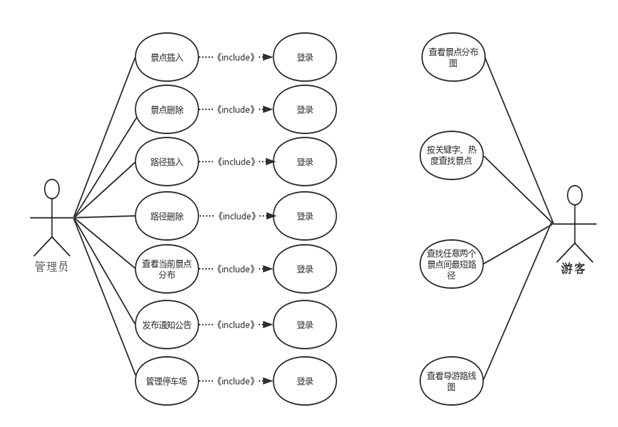1.3系统解决方案

1.4主要工作

2.1游客部分的系统设计
2.1.1景区分布图的数据结构

typedef struct ArcNode {//ArcNode变量类型存储边的信息
float time;
int distance;
string destination;
ArcNode* nextArc;//指向下一顶点
} ArcNode;
typedef struct VNode {//存储景点的基本信息
string name;//景点名称
string intro;//景点简介
int popular;//景点欢迎度
bool rest_place;//有无休息区
bool washroom;//有无公厕
bool visible = false;
int edgeNum = 0;
} ALGraph;

2.1.2文件信息数据结构
info.txt——存储景区信息

spots.txt——存储景区路径

2.1.3输出邻接矩阵数据结构

void TravelSystem::OutputGraph() {//输出图的信息，输出为邻接矩阵
int total = this->graph.vetexNum;
int spots = new int[total];
int matrix = new int[totaltotal];
int current = 0;
for (int m = 0; m < total; m++) {
for (int n = 0; n < total; n++) {
matrix[m
total+n]=(m==n ? 0 : has_Path(graph.vertices[spots[m]].name , graph.vertices[spots[n]].name));
}//对角线上元素取0，
}
for (int m = 0; m <total; m++) {
cout << setw(6) << setiosflags(ios::left) << setfill(’ ‘)
<< graph.vertices[spots[m]].name << " ";
for (int n = 0; n< total; n++) {
cout << setw(5) << setiosflags(ios::left) << setfill(’ ')
<< matrix[m*total+n] << " ";
}
cout << endl;
}
}

2.1.4景点查找排序设计

void TravelSystem::search() {
string key;
int result = 0;
ALGraph& G = graph;
cout << endl << “请输入搜索关键词” << endl;
cin >> key;
size_t found1;
size_t found2;
cout << endl;
for (int i = 0; i < MAX_VERTEX_NUM; i++) {
if (G.vertices[i].visible == true) {
found1 = G.vertices[i].name.find(key);
found2 = G.vertices[i].intro.find(key);
if (found1 != string::npos || found2 != string::npos)
……
}

2.1.5最短路径与最短距离设计

void TravelSystem::ShortestPath(int* D, int* path, int* spots) {
int total = this->graph.vetexNum;
int u, v, w;
for (v = 0; v < total; v++) {
for (w = 0; w < total; w++) {
D[vtotal + w] = (has_Path(graph.vertices[spots[v]].name, graph.vertices[spots[w]].name));
if (D[v
total + w] < INFINITY) {
path[vtotal + w] = v;//对最短路径初始化为自身的前一个结点的序号
}
}
}
for (u = 0; u < total; u++) {
for (v = 0; v < total; v++) {
for (w = 0; w < total; w++) {
if (D[v
total + u] + D[utotal + w] < D[vtotal + w]) {
D[vtotal + w] = D[vtotal + u] + D[utotal + w];
path[v
total + w] = u;
}
}
}
}
}

2.1.6查看导游路线图设计

void Traceablepath (Graph g)
{
(1)选择g的任一顶点r；
(2)用最小生成树算法找出带权图g的一棵以r为根的最小生成树T；
(3)前序遍历树T得到的顶点表L；
(4)将r加到表L的末尾，按表L中顶点次序组成回路H，作为计 算结果返回；
}

typedef struct {
int lowdist;//某顶点与当前生成树的顶点之间的最小权值
} MiniEdge;//Prime算法辅助结构体

2.2管理员部分的系统设计
2.2.1景点插入与删除设计

……//省略部分代码，仅保留关键代码
ALGraph& G = ts->graph;
G.vertices[post].visible = false;//不是直接删除而是将可见性设为0
G.IsFull[post] = 0;//定义该位置没满，下次输入的时候可以把该结点的信息覆盖
for (int i = 0; i<MAX_VERTEX_NUM; i++) {
if (G.vertices[i].visible == true) {
ts->delete_path(i, spot);//删除与该结点相关的边
}
}
ArcNode* temp;
while (prev != NULL && prev->nextArc != NULL) {
temp = prev->nextArc;
prev->nextArc = temp->nextArc;
delete temp;
}
delete prev;
G.vetexNum–;
……
}

2.2.2路径的插入与删除

2.2.3通知公告功能设计

2.3停车场系统设计
2.3.1停车场停车信息显示设计

2.4系统的关键类图设计如下：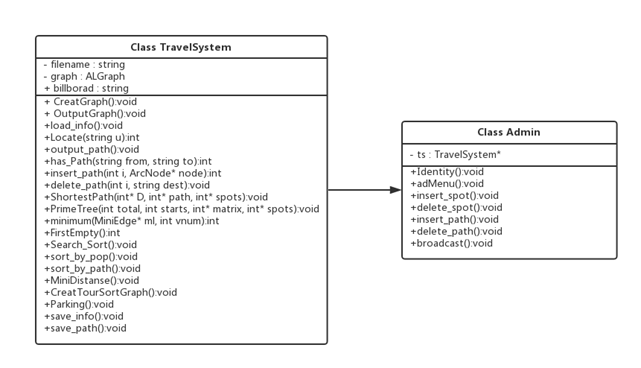3.1 景区路线图的初始化实现

3.2管理员管理景区实现

3.3停车场系统的实现

4.1管理员功能测试

4.1.1景点插入功能测试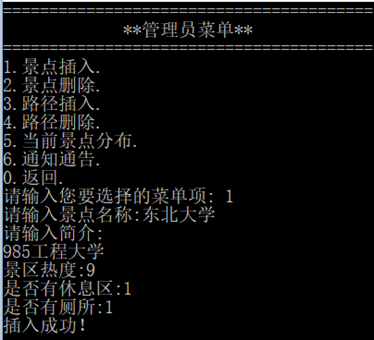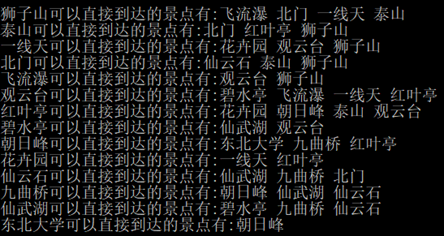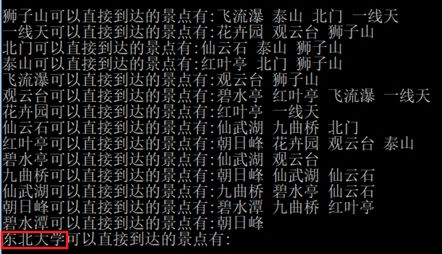4.1.2景点删除功能测试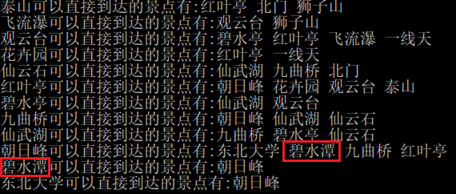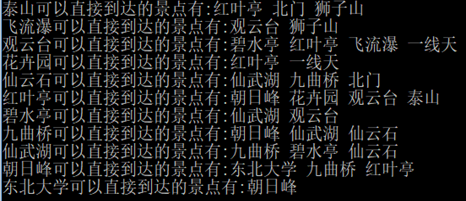4.2游客功能测试
4.2.1输出景点邻接矩阵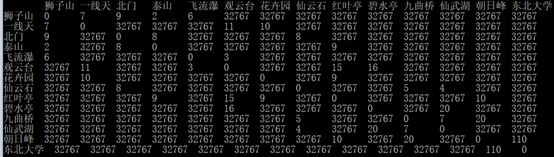4.2.2最短路径查询4.3停车场系统功能测试
4.3.1车辆到达登记与车位分配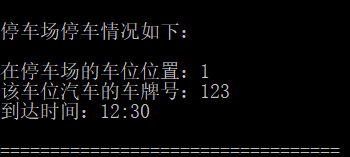4.3.2车辆离开与价格结算：5.1实验心得与体会

5.2系统实现的功能

5.3系统创新点

5.4系统待解决问题